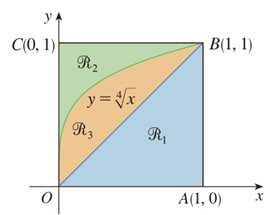Chapter 5.2, Problem 20E

Chapter
Section
Textbook Problem

# Refer to the figure and find the volume generated by rotating the given region about the specified line.ℛ 1 about OC

To determine

To find:

The volume generated by rotating the region R1 about the line  OC by using the figure.

Explanation

1) Concept:

i. If the cross section is a washer with the inner radius rin and the outer radius rout, then the area of the washer is obtained by subtracting the area of the inner disk from the area of the outer disk.

ii. The volume of the solid revolution about the y-axis is

V= abA(y)dy

2) Given:

Figure:

3) Calculation:

Here, the line OB appears to be the line y=x and the line OC appears to be the line  x=0.

Rotating R1 about OC that is the line  x=0, the cross section is perpendicular to the y -axis and it is a washer with the inner radius  y and the outer radius 1.

From figure, the solid lies between  y=0 and  y=1

### Still sussing out bartleby?

Check out a sample textbook solution.

See a sample solution

#### The Solution to Your Study Problems

Bartleby provides explanations to thousands of textbook problems written by our experts, many with advanced degrees!

Get Started